# Sine and Cosine GraphsPage 1

#### WATCH ALL SLIDES

Slide 1Graphing Sine and Cosine

Slide 2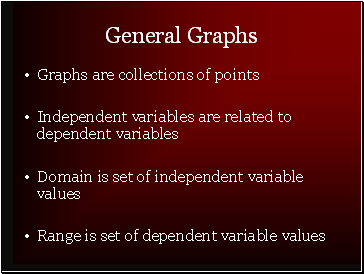## General Graphs

Graphs are collections of points

Independent variables are related to dependent variables

Domain is set of independent variable values

Range is set of dependent variable values

Slide 3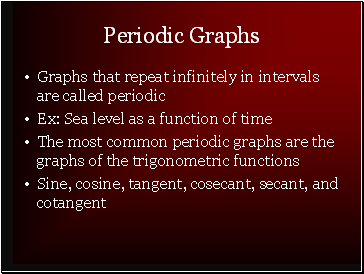## Periodic Graphs

Graphs that repeat infinitely in intervals are called periodic

Ex: Sea level as a function of time

The most common periodic graphs are the graphs of the trigonometric functions

Sine, cosine, tangent, cosecant, secant, and cotangent

Slide 4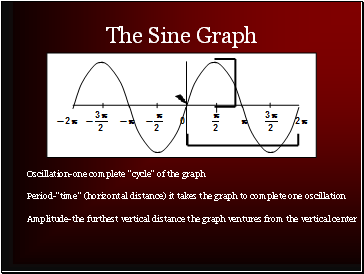## The Sine Graph

Oscillation-one complete “cycle” of the graph

Period-”time” (horizontal distance) it takes the graph to complete one oscillation

Amplitude-the furthest vertical distance the graph ventures from the vertical center

Slide 5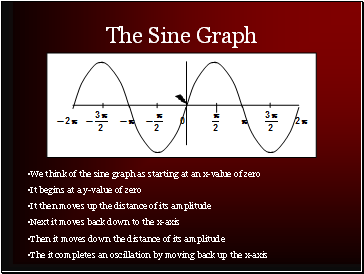The Sine Graph

We think of the sine graph as starting at an x-value of zero

It begins at a y-value of zero

It then moves up the distance of its amplitude

Next it moves back down to the x-axis

Then it moves down the distance of its amplitude

The it completes an oscillation by moving back up the x-axis

Slide 6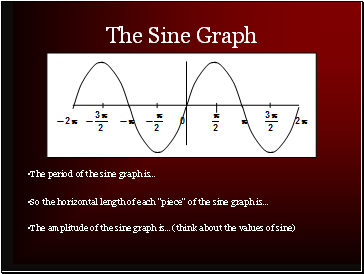The Sine Graph

The period of the sine graph is…

So the horizontal length of each “piece” of the sine graph is…

The amplitude of the sine graph is…(think about the values of sine)

Slide 7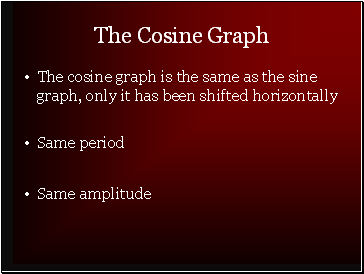The Cosine Graph

The cosine graph is the same as the sine graph, only it has been shifted horizontally

Same period

Same amplitude

Slide 8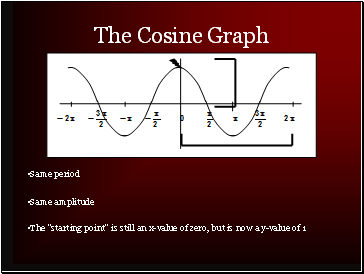## The Cosine Graph

Same period

Same amplitude

The “starting point” is still an x-value of zero, but is now a y-value of 1

Slide 9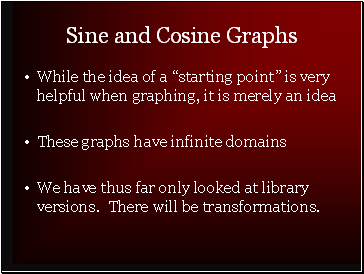## Sine and Cosine Graphs

While the idea of a “starting point” is very helpful when graphing, it is merely an idea

These graphs have infinite domains

We have thus far only looked at library versions. There will be transformations.